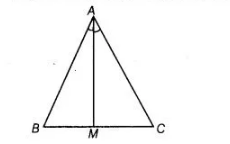# M is a point on side BC of a triangle`
Question:

M is a point on side BC of a triangle ABC such that AM is the bisector of ∠BAC. Is it true to say that perimeter of the triangle is greater than 2AM? Give

Solution:

Yes, in $\triangle A B C, M$ is a point of side $B C$ such that $A M$ is the bisector of $\angle B A C$.

$\ln \triangle A B M, \quad A B+B M>A M \quad \ldots$ (i)

[sum of two sides of a triangle is greater than the third side]

$\ln \triangle A C M$ $A C+C M>A M$ ... (ii)

[sum of two sides of a triangle is greater than the third side]On adding Eqs. (i) and (ii), we get

$(A B+B M+A C+C M)>2 A M$

$\Rightarrow \quad(A B+B M+M C+A C)>2 A M$

$\Rightarrow \quad A B+B C+A C>2 A M \quad[\because B C=B M+M C]$

$\therefore \quad$ Perimeter of $\triangle A B C>2 A M$